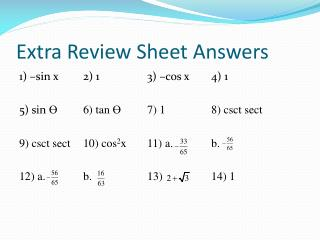# Extra Review Sheet Answers - PowerPoint PPT PresentationDownload PresentationDownload Presentation- - - - - - - - - - - - - - - - - - - - - - - - - - - E N D - - - - - - - - - - - - - - - - - - - - - - - - - - -
##### Presentation Transcript

1. Extra Review Sheet Answers 1) –sin x 2) 1 3) –cos x 4) 1 5) sin Ө 6) tan Ө 7) 1 8) csct sect 9) csct sect 10) cos2x 11) a. b. 12) a. b. 13) 14) 1

2. 15) a. b. 16) a. b. 17) 18) 19) 20) a. b. c.

3. Basic Idea: • We will play 3 rounds then a final bonus round • Each round contains 3 questions • You will be given the topics of these 3 questions • You will assign point values to these questions • You get the question correct, you earn those points • Bonus round is an open wager • Team(s) with highest points will be awarded delicious prizes

4. Round One: 1, 3, and 5 Points 1) Sum & Difference 2) Half-Angles 3) Simplify Using Identities

5. Round 1 Problems 1) Use a sum or difference formulas to find the exact value of sin 75̊ 2) Use a half-angle to find the exact value of cos 22.5̊ 3) Simplify:

6. Round Two: 2, 6, and 10 points 1) Simplify Using Identities 2) Double Angles 3) Sum & Difference

7. Round 2 Problems 1) Simplify: cos3x + cosx sin2x 2) Given: cot Ө = 2, cosӨ < 0, find the exact value of sin 2 Ө 3) Simplify:

8. Round 3: 3, 9, 15 points 1) Double Angles 2) Simplify Using Identities 3) Sum & Difference

9. Round 3 Problems 1) Use a double angle to find the exact value of 2) Simplify: 3) Use sum or difference formulas tofind the exact value of

10. Bonus Round: Make a Wager

11. αlies in quadrant II, and  lies in quadrant I Find the exact value of cos (α + )